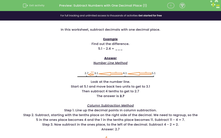# Practise Subtracting Numbers with One Decimal Place

In this worksheet, students subtract a mix of numbers with one decimal place. Two methods are shown.Key stage:  KS 2

Curriculum topic:   Number: Fractions, Decimals and Percentages

Curriculum subtopic:   Use up to Three Decimal Places

Popular topics:   Decimals worksheets

Difficulty level:#### Worksheet Overview

In this worksheet, we will be looking at how to subtract decimals with one decimal place.

Example

Find out the difference:

5.1 - 2.4 = ___

Number Line MethodLook at the number line.

Start at 5.1 and move back 2 ones to get to 3.1

Then subtract 4 tenths to get to 2.7

Column Subtraction Method

Step 1. Line up the decimal points in column subtraction.
Step 2. Subtract, starting with the tenths place on the right side of the decimal. We need to exchange, so the 5 in the ones place becomes 4 and the 1 in the tenths place becomes 11. Subtract 11 - 4 = 7.
Step 3. Now subtract in the ones place, to the left of the decimal. Subtract 4 - 2 = 2.